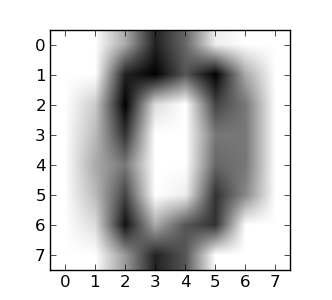# 1. Statistical learning: the setting and the estimator object in the scikit-learn¶

## 1.1. Datasets¶

The scikit-learn deals with learning information from one or more datasets that are represented as 2D arrays. They can be understood as a list of multi-dimensional observations. We say that the first axis of these arrays is the samples axis, while the second is the features axis.

A simple example shipped with the scikit: iris dataset

```>>> from scikits.learn import datasets
>>> data = iris.data
>>> data.shape
(150, 4)
```

It is made of 150 observations of irises, each described by 4 features: their sepal and petal length and width, as detailed in iris.DESCR.

When the data is not intially in the (n_samples, n_features) shape, it needs to be preprocessed to be used by the scikit.

An example of reshaping data: the digits datasetThe digits dataset is made of 1797 8x8 images of hand-written digits

```>>> digits = datasets.load_digits()
>>> digits.images.shape
(1797, 8, 8)
>>> import pylab as pl
>>> pl.imshow(digits.images, cmap=pl.cm.gray_r)
<matplotlib.image.AxesImage object at ...>
```

To use this dataset with the scikit, we transform each 8x8 image in a feature vector of length 64

```>>> data = digits.images.reshape((digits.images.shape, -1))
```

## 1.2. Estimators objects¶

Fitting data: The core object of the scikit-learn is the estimator object. All estimator objects expose a fit method, that takes a dataset (2D array):

```>>> estimator.fit(data)
```

Estimator parameters: All the parameters of an estimator can be set when it is instanciated, or by modifying the corresponding attribute:

```>>> estimator = Estimator(param1=1, param2=2)
>>> estimator.param1
1
```

Estimated parameters: When data is fitted with an estimator, parameters are estimated from the data at hand. All the estimated parameters are attributes of the estimator object ending by an underscore:

```>>> estimator.estimated_param_
```